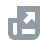Pay with
Markets
NFT
New
English
USDAccount FunctionsTutorialBinance Fan TokenBinance EarnCrypto Deposit/WithdrawalCrypto Derivatives
Futures Contracts
Introduction to Binance Futures
USDⓈ-M Futures Contracts
COIN-M Futures Contracts
Futures Guide
Cross Collateral
Hedge Mode
Types of Orders
Binance Futures Welcome Bonus Coupon
Futures Service Agreement
Binance Futures Related Programs
Index
Portfolio Margin
Options
Leveraged TokensFinanceAPISecurityOther TopicsNFTVIP

# Binance Portfolio Margin Account Maintenance Margin Ratio Calculation

2022-04-18 06:07
Under the Binance Portfolio Margin Program, the Maintenance Margin amount and ratio are calculated on a unified basis. For this reason, the initial margin requirement and position leverage are no longer required.
The Unified Maintenance Margin Ratio of the Binance Portfolio Margin account is defined as uniMMR.

## Details of the uniMMR calculation:

Unified Account Equity means the the sum of (1) the account balance of your nominated Futures Accounts, (2) unrealized profits (if any) minus any unrealized losses (if any) in respect of each position of your USD-M Futures and COIN-M Futures, (3) Total Assets Value minus Total Liability and Outstanding Interest of your Margin Account, when converted to US dollars.
Unified Maintenance Margin Amount means the sum of the total amount of Maintenance Margin or the Margin required to maintain margin levels, which is required to be held across all nominated Futures Accounts and Cross Margin Accounts when converted to US dollars.

## Collateral Calculation and Liquidation Example

Suppose user A has the following margin assets and loan and the margin leverage used is 3x cross-margin.
SpotMM = (Loan*MMR) / (1-MMR), while MMR = marginMM/marginAsset= 1-1/1.1 with 3x leverage.
Let’s calculate user A’s Equity and MM (Maintenance Margin):
Meanwhile, user A has the following Futures positions and assets in the USDⓈ-M Futures and COIN-M Futures accounts.
Let’s calculate user A’s Equity and MM:
Let’s combine the above calculation to get the Unified Account Equity and Unified Maintenance Margin Amount:
Unified Account Equity = (6,186*0.99*1.001) + (0.11*40,000*0.95) + (5*2,100*0.95) = 20,285.26
Unified Maintenance Margin Amount = (18.4*1.001) + (0.00525*40,000) + (1.5*2,100) = 3,378.41
uniMMR = (20,285.26/3,378.41) * 100% = 600.44%